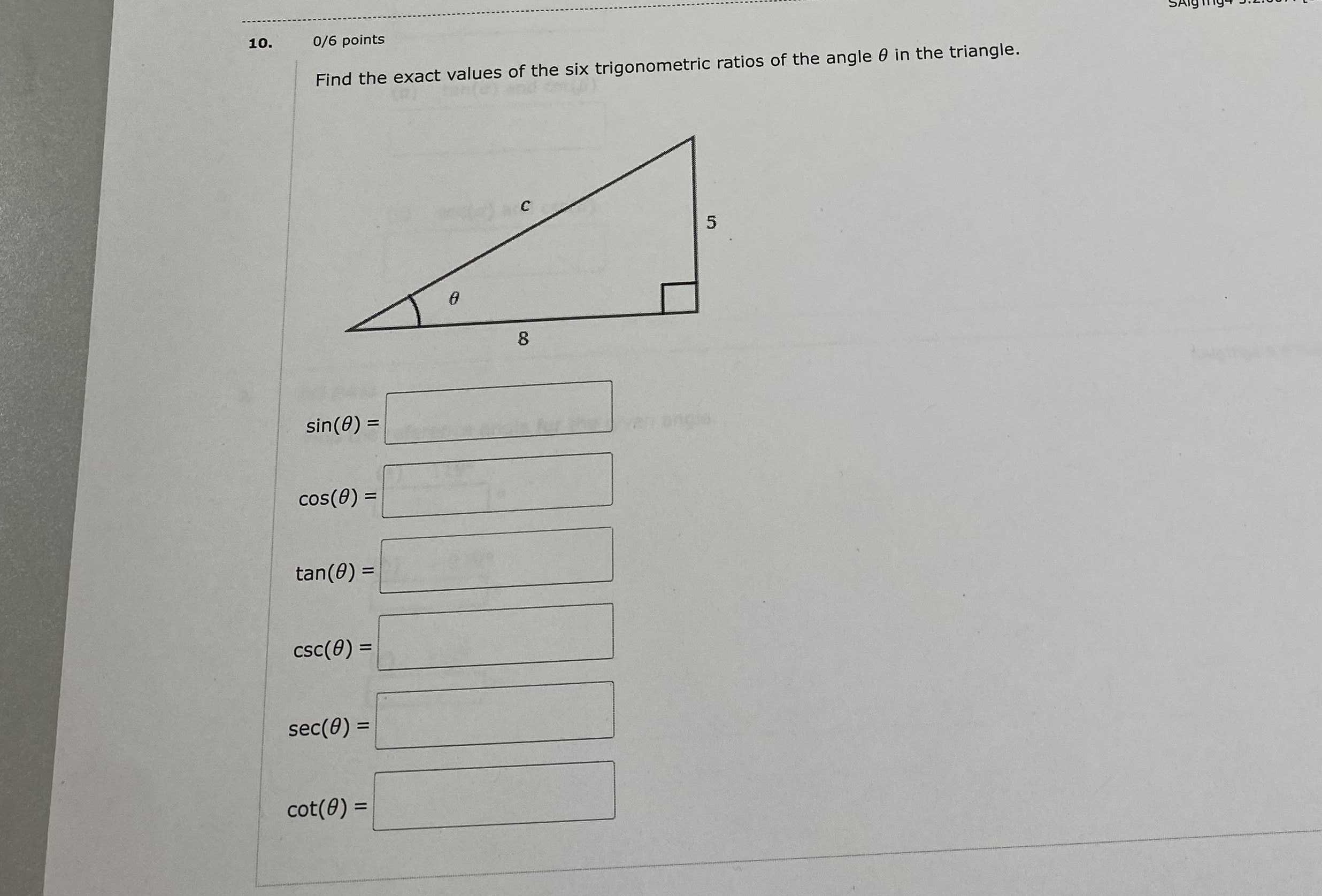### Still have math questions?

Trigonometry
QuestionFind the exact values of the six trigonometric ratios of the angle $$\theta$$ in the triangle. $$\sin ( \theta ) =$$

$$\cos ( \theta ) =$$

$$\tan ( \theta ) =$$

$$\csc ( \theta ) =$$

$$\sec ( \theta ) =$$

$$sin(\theta)= \frac{5}{\sqrt{89}}\\cos(\theta)= \frac{8}{\sqrt{89}}\\tan(\theta)= \frac{5}{8}\\csc(\theta)= \frac{\sqrt{89}}{5}\\sec(\theta)= \frac{\sqrt{89}}{8}\\cos(\theta)= \frac{8}{5}$$